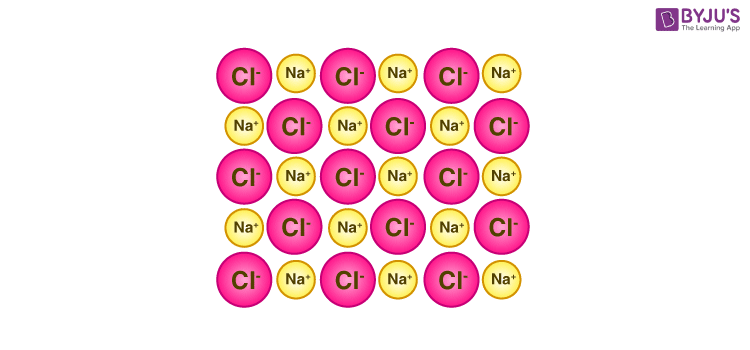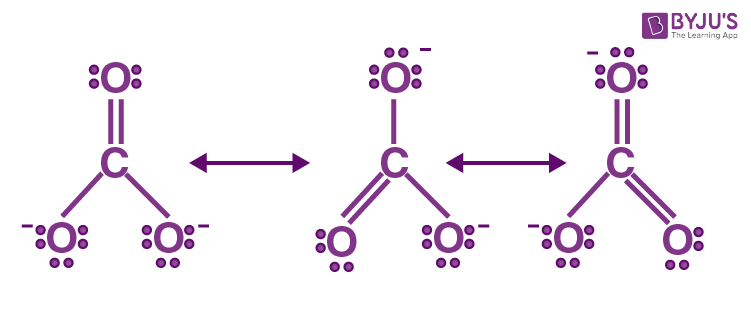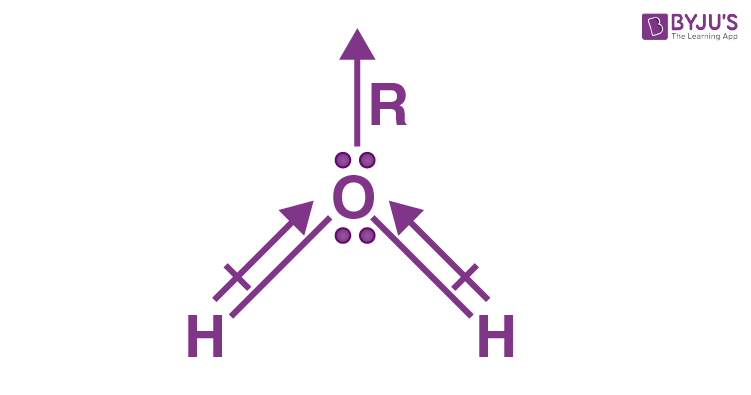Jet Set Go! All about Aeroplanes Jet Set Go! All about Aeroplanes

# Lattice Energy Questions

The strength of the ionic bonds in an ionic compound is measured by lattice energy. It reveals information about the volatility, solubility, and hardness of ionic solids. It is impossible to directly measure the lattice energy of an ionic solid. The Born-Haber cycle can, however, be used to estimate it. This amount is commonly given as kilojoules per mole (kJ/mol).

A sodium chloride molecule’s crystal structure is depicted below.The lattice energy is the energy required for the next reaction to take place in this ionic molecule.

NaCl(s) → Na+(g) + Cl(g)

In this case, the energy required to separate 1 mole of sodium chloride into gaseous Na+ and Cl ions is 786 kilojoules.

Definition: The difference in energy between the expected experimental value for the energy of the ionic solid and the actual value obtained is known as lattice energy. This is the energy difference between the energy of the individual gaseous ions and the energy of the ionic solid.

## Lattice Energy Chemistry Questions with Solutions

Q1: Many ionic compounds have some covalent ability due to

a) electron polarization

b) ion polarization

c) charge polarization

d) proton polarization

Q2: Lithium Fluoride’s lattice energy to Magnesium oxide is

a) greater

b) lesser

c) same

d) never known

Q3: The value of lattice energy is affected by

a) size and charge of ions

b) size of ions only

c) charge of ions only

d) mass of ions

Answer: a) size and charge of ions

Q4: Greater polarization of carbonate ion will result in weak

a) C=C bond

b) C-H bond

c) C-C bond

d) H-H bond

Q5: Lattice energy is inversely proportional to sum of radii of

a) cation only

b) anion only

c) anion and cation

d) ions

Q6: Define Lattice energy. How is Lattice energy influenced by (i) Charge on the ions (ii) Size of the ions?

The energy generated when one mole of crystalline solid is formed by the combination of oppositely charged ions is known as lattice energy.

• As the magnitude of an ion’s charge increases, the force of interionic attraction increases, and the value of Lattice energy increases.
• The smaller the ions, the shorter the antinuclear distance and consequently the higher the Lattice energy.

Q7: Which are the factors that contribute to lattice energy of an ionic compound?

The strength of the ionic bonds in an ionic compound is measured by lattice energy. It reveals information about ionic materials’ volatility, solubility, and hardness, among other things.

• The distance between the ions has an inverse relationship with the lattice energy of an ionic compound. The weaker the electrostatic forces binding ions together in a lattice, the lower the lattice energy.
• The ionic lattice of smaller atoms has smaller interatomic distances and stronger binding forces. As a result, the smaller the constituent ions are, the higher the ionic solid’s lattice energy.

Q8: What is the difference between lattice enthalpy and lattice energy

LATTICE ENTHALPY

The strength of the forces between the ions in an ionic solid is measured by lattice enthalpy. The stronger the forces, the greater the lattice enthalpy.

LATTICE ENERGY

The energy required to separate a mole of an ionic solid into gaseous ions is known as lattice energy. Although lattice energy cannot be measured experimentally, it can be calculated or estimated using electrostatics or the Born-Haber cycle.

The following equation can be used to represent the molar lattice energy of an ionic crystal in terms of molar lattice enthalpy, pressure, and volume change:

ΔGU = ΔGH – pΔVm

Where:

• ΔGU denotes the molar lattice energy.
• ΔGH denotes the molar lattice enthalpy.
• ΔVm is the change in volume (per mole).
• p is the pressure.

Q9: Mention the general characteristics of Ionic Compounds.

(i) Physical State: They are crystalline solids with a crystal lattice structure. Unlike other gaseous molecules such as H2, N2, 02, and Cl2, Ionic compounds do not exist as single molecules.

(ii) Melting and boiling points: Ionic compounds have high melting and boiling points due to the high interionic force.

(iii) Solubility: They dissolve in polar solvents like water but not in organic solvents like benzene, CCl4, and others.

(iv) Electrical conductivity: They are poor conductors of electricity in their solid state, but they conduct electricity when melted or dissolved in water.

(v) Ionic reactions: Ionic compounds produce ions in the solution, which react quickly with oppositely charged ions.

Q10: Explain the important aspects of resonance with reference to the CO32− ion.

All C-O bonds in CO32− are equal, according to experimental results.

As a result, using a single Lewis structure with one double bond and two single bonds to represent CO32− ion is inefficient.

As a result, the resonance structures of CO32− are:Q11: What are the practical applications of Lattice Enthalpies?

Lattice energy is used to evaluate electron and fluoride relationships, to estimate standard enthalpies formation, and to measure the strength of ionic solids. An in-depth explanation is –

When a group of ions come together to form a molecule, the Lattice energy factor helps in determining how much energy is released.

It’s primarily used to figure out how strong ionic compounds or solids are. Ionic solids or compounds typically have a high lattice energy, which allows them to achieve greater stability and makes it difficult to break their bond.

Q12: Although both CO2 and H2O are triatomic molecules, the shape of the H2O molecule is bent while that of CO2 is linear. Explain this on the basis of the dipole moment.

CO2 has a dipole moment of 0 according to experimental observations. And it’s only possible if the molecule’s structure is linear, because the dipole moments of the C-O bond are equal and opposite, nullifying each other.Thus, resultant μ = 0

The dipole moment of H2O is 1.84 D. Since the dipole moments of the O-H bond are unequal, the value of dipole moments indicates that the structure of the water molecule is bent.Q13: How does charge on the ion effect Lattice Enthalpy?

Because of the electrostatic attraction between the particles in the grid gem, they are drawn in. Given that this power is directly proportional to the magnitude of charge, the larger the charge, the more grounded in the cross-section.

Potassium Chloride and Calcium Chloride, for example, have a similar gem grid game plan, but the last option’s cross-section enthalpy is higher than the first. This is because calcium particles have 2+ charges while potassium particles only have 1+ charge, and because we know that electrostatic power of interest is directly proportional to charge, calcium chloride has a more grounded power.

Q14: Mention the difference between Electronegativity and Electron affinity.

Sr. No

Electronegativity

Electron Affinity

1

Electronegativity is the tendency of an atom in a chemical compound to attract shared pairs of electrons.

The electron gain enthalpy of an isolated gaseous atom is its inclination to gain electrons.

2

It differs depending on the element to which it is bonded.

It is unaffected by the element to which it is attached.

3

It is not constant for any element.

It is constant for an element.

4

It is not a measurable quantity.

It is a measurable quantity.

Q15: Which out of NH3 and NF3 has higher dipole moment and why?

The central atom in NF3 and NH3 is the N- atom.

The central atom has one lone pair and three bond pairs. As a result, the shape for both is AB3E, or pyramidal.

NF3 should have a higher dipole moment than NH3 because the F-atom has a higher electronegativity than the H-atom. However, the dipole moment of NH3 is 1.46D, higher than that of NF3, which is 0.24D.

The directions of individual bond dipole moments in NF3 and NH3 demonstrate this.

Since both N-H bonds point in the same direction, they add to the bond moment of the lone pair, whereas N-F bonds point in the opposite way, they cut the bond moment of the lone pair in half.

As a result, NH3 has a greater dipole moment than NF3.

## Practise Questions on Lattice Energy

Q1: Explain the formation of a chemical bond.

Q2: Write the favourable factors for the formation of ionic bonds.

Q3: How to Calculate Lattice Enthalpy?

Q4: Although geometries of NH3 and H2O molecules are distorted tetrahedral, the bond angle in water is less than that of ammonia. Discuss.

Q5: Arrange the bonds in order of increasing ionic character in the molecules: LiF, K2O, N2, SO2, and ClF3.

Click the PDF to check the answers for Practice Questions.

## Solid State | Concept Visualised | Crystal Lattice and Unit Cell |